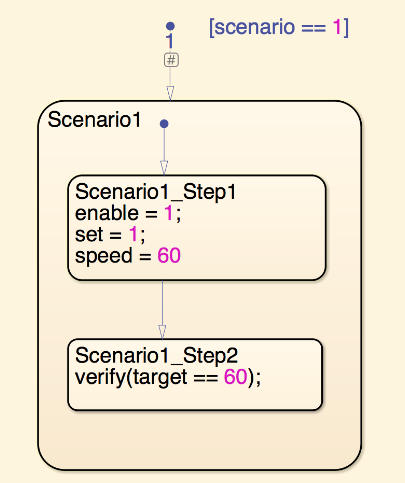# verify

Assess logical expression and automatically log result

## Syntax

``verify(expression)``
``verify(expression,errorMessage)``
``verify(expression,identifier,errorMessage)``

## Description

example

````verify(expression)` evaluates a scalar logical `expression` to `true` or `false`.```

example

````verify(expression,errorMessage)` returns the specified error message for the failed `verify` statement. If you run the test in the Test Manager, the error message appears in the simulation log. If you run the test outside the Test Manager, the message appears in the Diagnostic Viewer. To format the error message, you can use any `sprintf` format, except strings and chars in Stateflow® charts.```

example

````verify(expression,identifier,errorMessage)` uses the `identifier` as a label for the test results. The `identifier` is used as the signal label in the Test Manager. If you run the test outside the Test Manager, the label appears in the Simulation Data Inspector or, for a failure, in the Diagnostic Viewer. The identifier is a string that has at least two colon-separated MATLAB® identifiers.```

## Examples

expand all

```verify(x > y && z > 10) ```

If this `verify` statement fails, it returns an error message that lists the values of `x`, `y`, and `z`.

```verify(x > y && z > 10,'x, y, and z are %d,%d,%d',x,y,z) ```

The result of this `verify` statement is prefaced by the label, `TestReq1:bothGreater` and, if the test fails, the error message.

```verify(x > y && z > 10,'TestReq1:bothGreater',... 'x, y, and z are %d,%d,%d',x,y,z) ```

The second step in the Scenario1 state of this Stateflow chart verifies that the target equals 60.## Tips

• You can use `verify` statements in Test Sequence and Test Assessment blocks and in Stateflow charts. A Stateflow license is required to use a chart. `verify` statements in charts are supported in the same locations, execution modes, and for the same code generation targets as the Test Sequence block.

• You cannot use `verify` statements in:

• Test Sequence blocks that use continuous-time updating. Test Sequence block data can depend on factors such as the solver step time. Continuous-time updating can cause differences in when block data and `verify` statements update, which can lead to unexpected `verify` statement results. If your model uses continuous time and you use `verify` statements in a Test Sequence or Test Assessment block, consider explicitly setting a discrete block sample time.

• Moore, Mealy, Discrete Event, or continuous charts

• Charts that use C as the action language

• Bind actions in a chart

• Transition or condition actions in a chart

• MATLAB functions, graphical functions, or truth tables in a chart

• MATLAB Function or Truth Table blocks

• Rapid Accelerator mode simulations

• Code generation targets other than Simulink® Real-Time™ and HDL Verifier™

• Standalone Stateflow charts

• If you use parallel test execution to run your tests, then you cannot use the button for in the Test Manager to `verify` results.

• You cannot use `verify` as a condition immediately after `when` in a When decomposition because `verify` statements do not produce output. You can, however, use `verify` statements as actions in When decomposition steps. See Assess a Model by Using When Decomposition.

• When comparing floating-point data in `verify` statements, consider the precision limitations associated with floating-point numbers. If you need to use floating-point data, define a tolerance for the verification. For example, instead of `verify(x == 5)`, verify `x` within a tolerance of 0.001:

`verify(abs(x-5) < 0.001)`